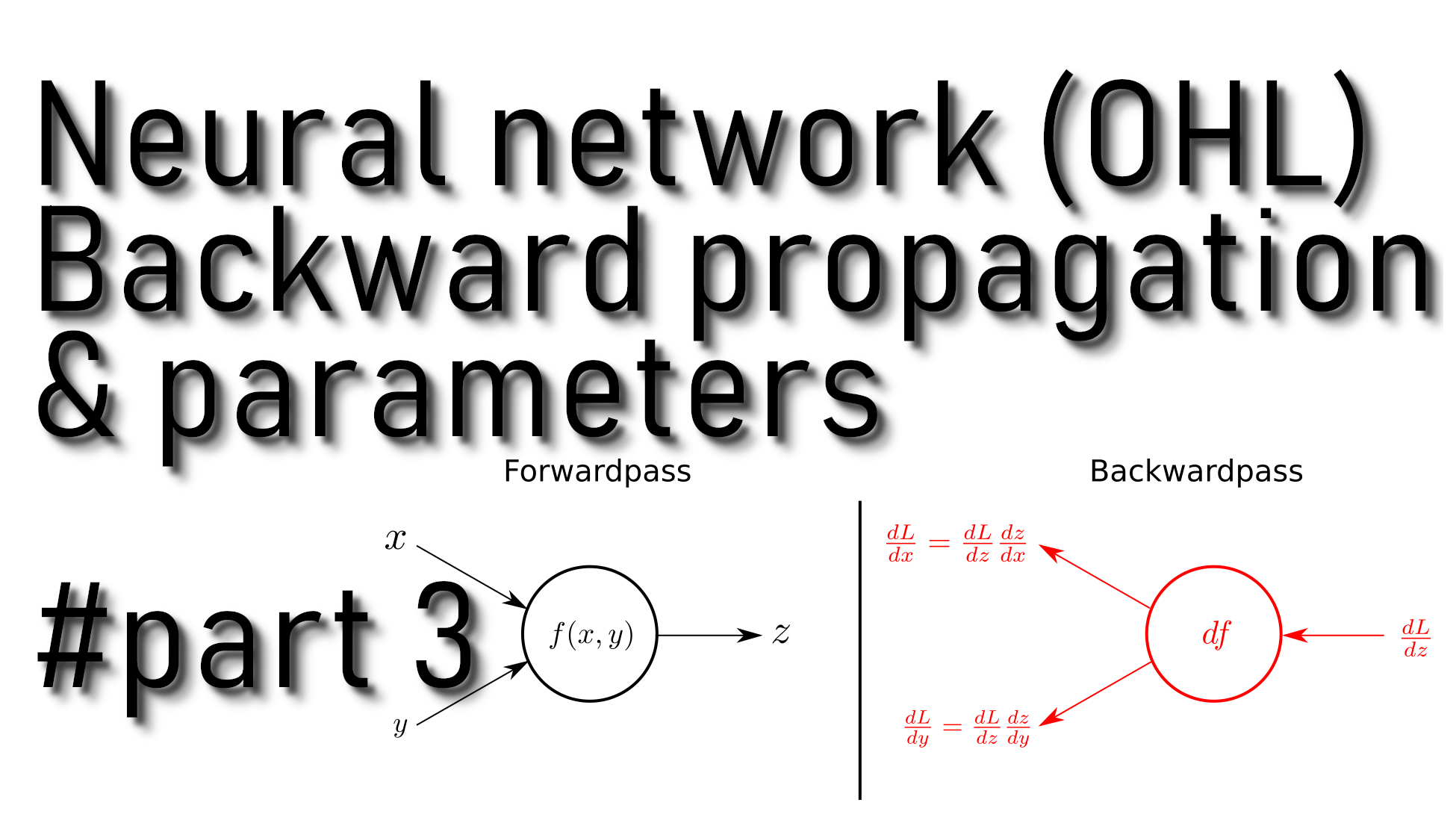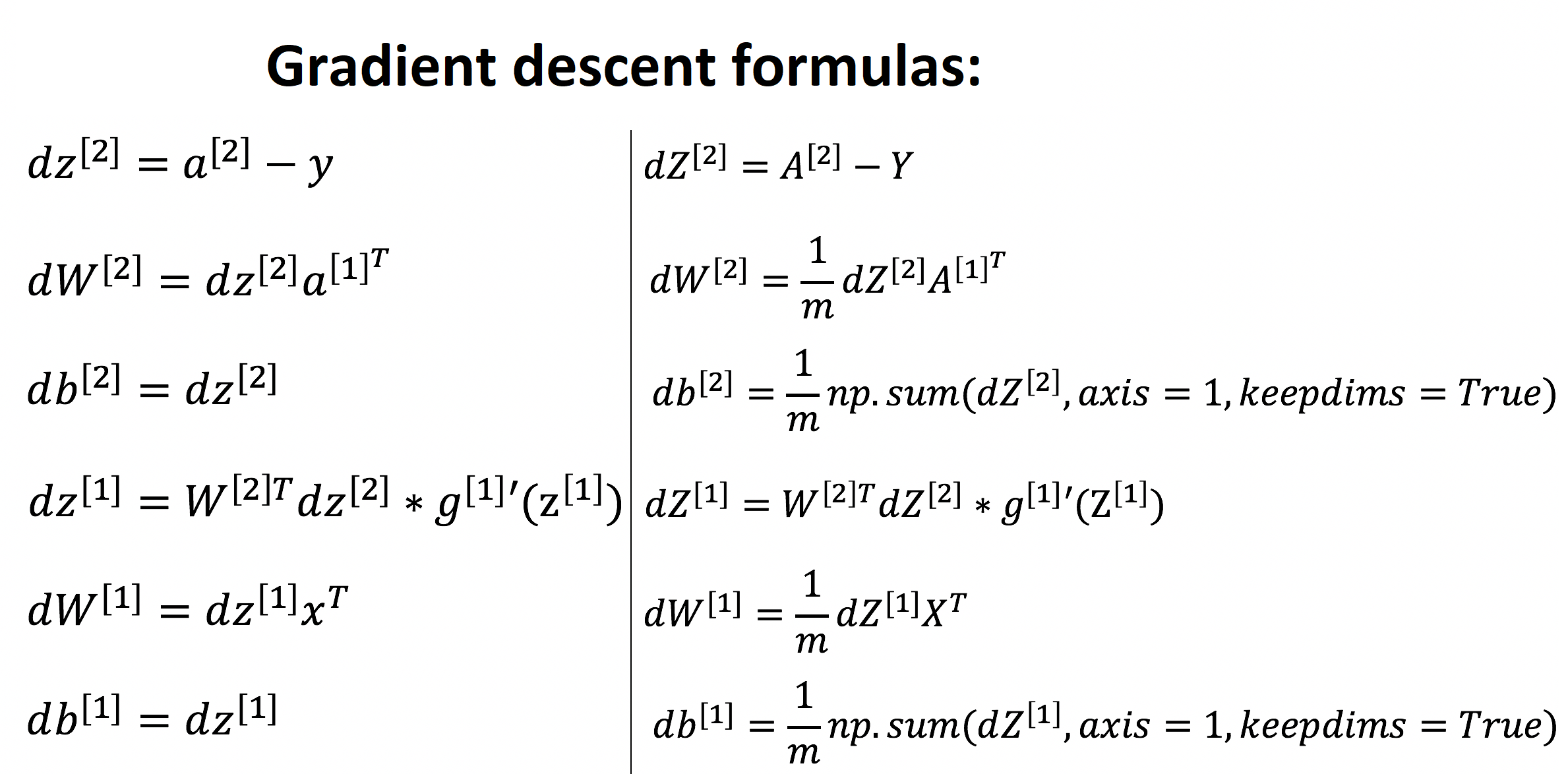# Neural Network Backward propagation and parameters update

## In this tutorial part, we'll write backward propagation and parameters update function using cache computed during the forward propagation

In our previous tutorial, we wrote forward propagation and cost functions. So next, we need to write a backpropagation function. For this, we'll use cache computed during the forward propagation.

Backpropagation is usually the hardest (most mathematical) part of deep learning. Here again, is the picture with six mathematical equations we'll use. We'll use six equations on the right of this image since we are building a vectorized implementation.## Code for our backward propagation function:

Arguments:

parameters - python dictionary containing our parameters;
cache - a dictionary containing "Z1", "A1", "Z2" and "A2";
X - input data of shape (input, number of examples);
Y - "true" labels vector of shape (1, number of examples).

Return:

grads - python dictionary containing our gradients with respect to different parameters.

``````def backward_propagation(parameters, cache, X, Y):
# number of example
m = X.shape

# Retrieve W1 and W2 from the "parameters" dictionary
W1 = parameters["W1"]
W2 = parameters["W2"]

# Retrieve A1 and A2 from "cache" dictionary
A1 = cache["A1"]
A2 = cache["A2"]

# Backward propagation for dW1, db1, dW2, db2
dZ2 = A2-Y
dW2 = 1./m*np.dot(dZ2, A1.T)
db2 = 1./m*np.sum(dZ2, axis = 1, keepdims=True)
dZ1 = np.dot(W2.T, dZ2) * (1 - np.power(A1, 2))
dW1 = 1./m*np.dot(dZ1, X.T)
db1 = 1./m*np.sum(dZ1, axis = 1, keepdims=True)

"db1": db1,
"dW2": dW2,
"db2": db2}

## Code for our update parameters function:

We'll implement the update rule using gradient descent. So we'll have to use (dW1, db1, dW2, db2) to update (W1, b1, W2, b2).

Arguments:

parameters - python dictionary containing our parameters;
learning_rate - learning rate of the gradient descent update rule;

Return:

parameters -- python dictionary containing your updated parameters

``````def update_parameters(parameters, grads, learning_rate = 0.1):
# Retrieve each parameter from the dictionary "parameters"
W1 = parameters["W1"]
W2 = parameters["W2"]
b1 = parameters["b1"]
b2 = parameters["b2"]

# Update rule for each parameter
W1 = W1 - dW1 * learning_rate
b1 = b1 - db1 * learning_rate
W2 = W2 - dW2 * learning_rate
b2 = b2 - db2 * learning_rate

parameters = {"W1": W1,
"b1": b1,
"W2": W2,
"b2": b2}

return parameters``````

## Full tutorial code:

``````import os
import cv2
import numpy as np
import matplotlib.pyplot as plt
import scipy

ROWS = 64
COLS = 64
CHANNELS = 3

#TRAIN_DIR = 'Train_data/'
#TEST_DIR = 'Test_data/'

#train_images = [TRAIN_DIR+i for i in os.listdir(TRAIN_DIR)]
#test_images =  [TEST_DIR+i for i in os.listdir(TEST_DIR)]

return cv2.resize(img, (ROWS, COLS), interpolation=cv2.INTER_CUBIC)

def prepare_data(images):
m = len(images)
X = np.zeros((m, ROWS, COLS, CHANNELS), dtype=np.uint8)
y = np.zeros((1, m))
for i, image_file in enumerate(images):
if 'dog' in image_file.lower():
y[0, i] = 1
elif 'cat' in image_file.lower():
y[0, i] = 0
return X, y

def sigmoid(z):
s = 1/(1+np.exp(-z))
return s
'''
train_set_x, train_set_y = prepare_data(train_images)
test_set_x, test_set_y = prepare_data(test_images)

train_set_x_flatten = train_set_x.reshape(train_set_x.shape, ROWS*COLS*CHANNELS).T
test_set_x_flatten = test_set_x.reshape(test_set_x.shape, -1).T

train_set_x = train_set_x_flatten/255
test_set_x = test_set_x_flatten/255
'''
#train_set_x_flatten shape: (12288, 6002)
#train_set_y shape: (1, 6002)

def initialize_parameters(input_layer, hidden_layer, output_layer):
# initialize 1st layer output and input with random values
W1 = np.random.randn(hidden_layer, input_layer) * 0.01
# initialize 1st layer output bias
b1 = np.zeros((hidden_layer, 1))
# initialize 2nd layer output and input with random values
W2 = np.random.randn(output_layer, hidden_layer) * 0.01
# initialize 2nd layer output bias
b2 = np.zeros((output_layer,1))

parameters = {"W1": W1,
"b1": b1,
"W2": W2,
"b2": b2}

return parameters

def forward_propagation(X, parameters):
# Retrieve each parameter from the dictionary "parameters"
W1 = parameters["W1"]
b1 = parameters["b1"]
W2 = parameters["W2"]
b2 = parameters["b2"]

# Implementing Forward Propagation to calculate A2 probabilities
Z1 = np.dot(W1, X) + b1
A1 =  np.tanh(Z1)
Z2 = np.dot(W2, A1) + b2
A2 = sigmoid(Z2)

# Values needed in the backpropagation are stored in "cache"
cache = {"Z1": Z1,
"A1": A1,
"Z2": Z2,
"A2": A2}

return A2, cache

def compute_cost(A2, Y, parameters):
# number of example
m = Y.shape

# Compute the cross-entropy cost
logprobs = np.multiply(np.log(A2),Y) +  np.multiply(np.log(1-A2), (1-Y))
cost = -1/m*np.sum(logprobs)

# makes sure cost is in dimension we expect, E.g., turns [] into 51
cost = np.squeeze(cost)

return cost

def backward_propagation(parameters, cache, X, Y):
# number of example
m = X.shape

# Retrieve W1 and W2 from the "parameters" dictionary
W1 = parameters["W1"]
W2 = parameters["W2"]

# Retrieve A1 and A2 from "cache" dictionary
A1 = cache["A1"]
A2 = cache["A2"]

# Backward propagation for dW1, db1, dW2, db2
dZ2 = A2-Y
dW2 = 1./m*np.dot(dZ2, A1.T)
db2 = 1./m*np.sum(dZ2, axis = 1, keepdims=True)
dZ1 = np.dot(W2.T, dZ2) * (1 - np.power(A1, 2))
dW1 = 1./m*np.dot(dZ1, X.T)
db1 = 1./m*np.sum(dZ1, axis = 1, keepdims=True)

"db1": db1,
"dW2": dW2,
"db2": db2}

def update_parameters(parameters, grads, learning_rate = 0.1):
# Retrieve each parameter from "parameters" dictionary
W1 = parameters["W1"]
W2 = parameters["W2"]
b1 = parameters["b1"]
b2 = parameters["b2"]

# Update rule for each parameter
W1 = W1 - dW1 * learning_rate
b1 = b1 - db1 * learning_rate
W2 = W2 - dW2 * learning_rate
b2 = b2 - db2 * learning_rate

parameters = {"W1": W1,
"b1": b1,
"W2": W2,
"b2": b2}

return parameters``````

## Conclusion:

So up to this point, we already wrote parameter initialization, forward propagation, backward propagation, cost, and parameters update functions. So in the next tutorial, we'll connect all of them into a model, and we'll start training neural networks with one hidden layer.